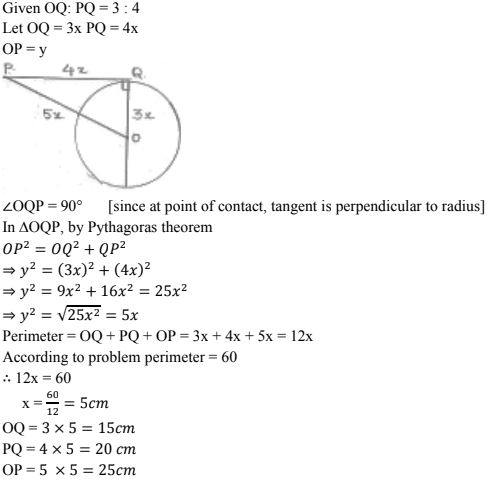# RD Sharma Solutions Chapter 10 Circles Exercise 10.2 Class 10 MathsChapter Name RD Sharma Chapter 10 Circles Book Name RD Sharma Mathematics for Class 10 Other Exercises Exercise 10.1 Related Study NCERT Solutions for Class 10 Maths

### Exercise 10.2 Solutions

1. If PT is a tangent at T to a circle whose center is O and OP = 17 cm, OT = 8 cm. Find the length of tangent segment PT.

Solution

OT = radius = 8 cm
OP = 17 cm
PT = length of tangent = ?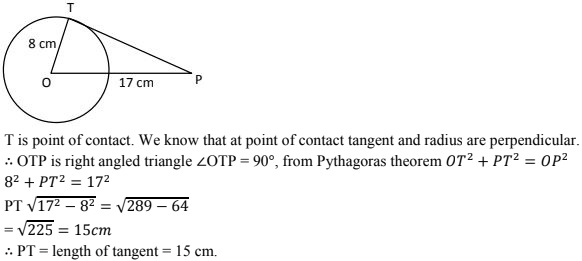2. Find the length of a tangent drawn to a circle with radius 5cm, from a point 13 cm from the center of the circle.

Solution

Consider a circle with center O.
A tangent is drawn at point P, such that line through O intersects it at Q, OB = 13cm.
Length of tangent PQ = ?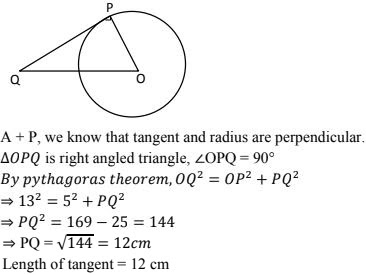3. A point P is 26 cm away from O of circle and the length PT of the tangent drawn from P to the circle is 10 cm. Find the radius of the circle.

Solution

Given OP = 26 cm
PT = length of tangent  = 10 cm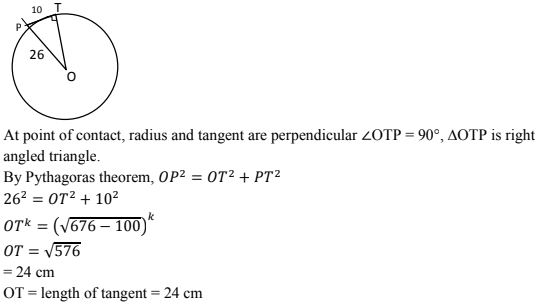4. If from any point on the common chord of two intersecting circles, tangents be drawn to circles, prove that they are equal.

Solution

Let the two circles intersect at points X and Y.
XY is the common chord.
Suppose 'A' is a point on the common chord and AM and AN be the tangents drawn A to the circle.
We need to show that AM = AN.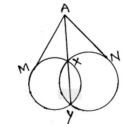In order to prove the above relation, following property will be used.
"Let PT be a tangent to the circle from an external point P and a secant to the circle through P intersects the circle at points A and B, then PT2 = PA × PB"
Now AM is the tangent and AXY is a secant
∴ AM2 = AX × AY ...(i)
AN is a tangent and AXY is a secant
∴ AN2 = AX × AY ...(ii)
From (i) & (ii), we have  AM2 = AN2
∴ AM = AN

5. If the quadrilateral sides touch the circle prove that sum of pair of opposite sides is equal to the sum of other pair.
Solution
Consider a quadrilateral ABCD touching circle with center O at points E, F, G and H as in figure.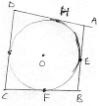We know that
The tangents drawn from same external points to the circle are equal in length.
1. Consider tangents from point A [AM ⊥ AE]
AH = AE ...(i)
2. From point B [EB & BF]
BF = EB ...(ii)
3. From point C [CF &  GC]
FC = CG  ...(iii)
4. From point D [DG  & DH]
DH = DG  ...(iv)
Adding (i), (ii), (iii), & (iv)
(AH + BF + FC + DH) = [(AC + CB) + (CG + DG)]
⇒ (AH + DH) + (BF + FC) = (AE + EB) + (CG + DG)
⇒ AD + BC = AB + DC  [from fig.]
Sum of one pair of opposite sides is equal to other.

6. If AB, AC, PQ are tangents in Fig. and AB = 5cm find the perimeter of ΔAPQ.
Solution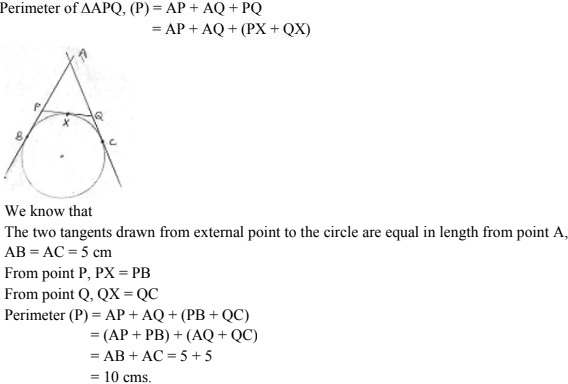7. Prove that the intercept of a tangent between two parallel tangents to a circle subtends a right angle at center.
Solution
Consider circle with center 'O' and has two parallel tangents through A & B at ends of diameter.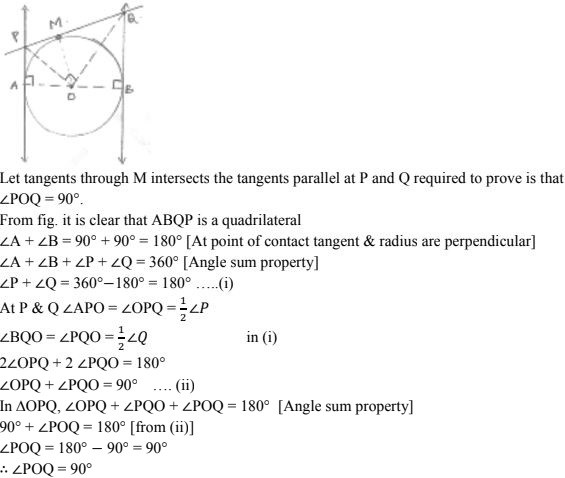8. In Fig below, PQ is tangent at point R of the circle with center O. If  ∠ TRQ = 30° . Find. ∠PRS.
Solution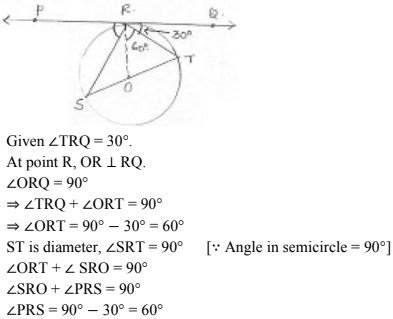9. If PA and PB are tangents from an outside point P. Such that PA = 10 cm and ∠APB  = 60°.  Find the length of chord AB.
Solution
AP = 10 cm
∠APB = 60°
Represented in the figure
We know that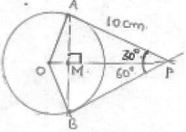A line drawn from center to point from where external tangents are drawn divides or bisects the angle made by tangents at that point ∠APO = ∠OPB = 1/2 × 60° = 30°
The chord AB will be bisected perpendicularly
∴ AB = 2AM
In ΔAMP,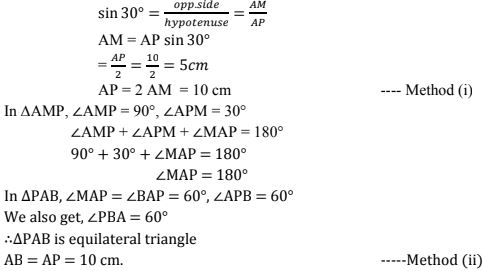10. From an external point P, tangents PA and PB are drawn to the circle with centre O. If CD is the tangent to the circle at point E and PA = 14 cm. Find the perimeter of ABCD.
Solution
PA = 14 cm
Perimeter of ΔPCD = PC + PD + CD = PC + PD + CE + ED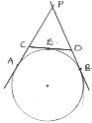We know that
The two tangents drawn from external point to the circle are equal in length.
From point P, PA = PB = 14 cm
From point C, CE = CA
From, point D, DB = ED
Perimeter = PC + PD + CA + DB
= (PC  + CA)  + (PD + DB)
= PA + PB = 14 + 14 = 28 cm.

11. In the fig. ABC is right triangle right angled at B such that BC = 6cm and AB = 8cm. Find the radius of its in circle.
Solution
BC = 6cm AB = 8cm
As ABC is right angled triangle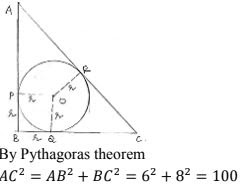AC = 10 cm
Consider BQOP ∠B = 90° ,
∠BPO = ∠OQB = 90° [At point of contact, radius is perpendicular to tangent]
All the angles = 90° & adjacent sides are equal
∴ BQOP is square BP = BQ = r
We know that
The tangents drawn from any external point are equal in length.
AP = AR = AB - PB = 8 - r
QC = RC = BC - BQ = 6 - r
AC = AR + RC ⇒ 10 = 8 - r + 6 - r
⇒ 10 = 14 - 2r
⇒ 2r = 4

12. From a point P, two tangents PA and PB are drawn to a circle with center O. If OP = diameter of the circle shows that ΔAPB is equilateral.
Solution
OP = 2r
Tangents drawn from external point to the circle are equal in length PA = PB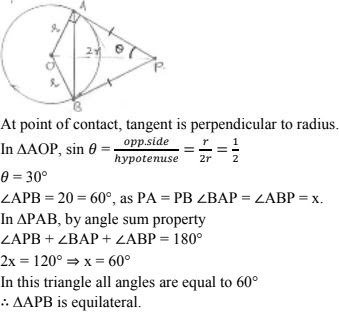13. Two tangent segments PA and PB are drawn to a circle with center O such that ∠APB = 120° . Prove that OP = 2AP.
Solution14. If ΔABC is isosceles with AB = AC  and C (0, 2) is the in circle of the ΔABC touching BC at L, prove that L, bisects BC.
Solution
Given ΔABC is isosceles AB = AC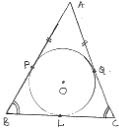We know that
The tangents from external point to circle are equal in length
From point A, AP = AQ
But AB = AC
⇒ AP + PB = AQ + QC
⇒ PB = PC ...(i)
From B, PB = BL  ...(ii)
from C, CL = CQ  ...(iii)
From (i), (ii) & (iii)
BL = CL
∴ L bisects BC.

15. In fig. a circle touches all the four sides of quadrilateral ABCD with AB = 6 cm, BC = 7 cm, CD = 4 cm. Find AD.
Solution
We know that the tangents drawn from any external point to circle are equal in length.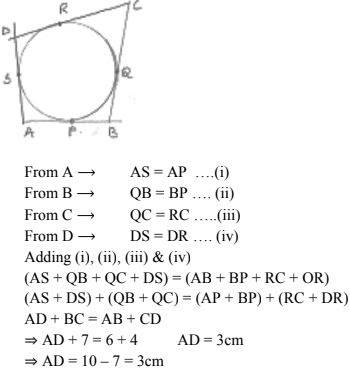16. Prove that the perpendicular at the point of contact to a circle passes through the center of the circle.
Solution
We know that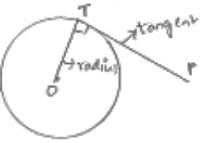The at point of contact, the tangent is perpendicular to the radius is line from center to point on circle. Therefore, perpendicular to tangent will pass through center of circle.

17. In fig.. O is the center of the circle and BCD is tangent to it at C. Prove that ∠BAC + ∠ACD = 90°
Solution
Given
O is center of circle
BCD is tangent.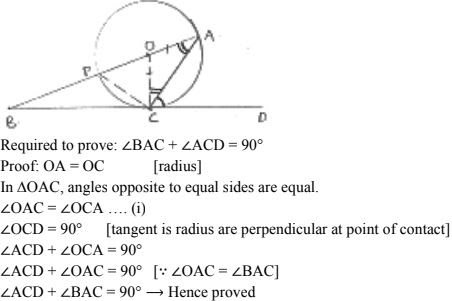18. Two circles touch externally at a point P from a point T on the tangent at P, tangents TQ and TR are drawn to the circles with points of contact Q and E respectively. Prove that TQ = TR.
Solution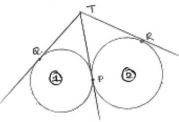Let the circles be represented by (i) & (ii) respectively
TQ, TP are tangents to (i)
TP, TR are tangents to (ii)
We know that
The tangents drawn from external point to the circle will be equal in length.
For circle (i), TQ = TP ...(i)
For circle (ii), TP = TR ...(ii)
From (i) & (ii) TQ = TR

19. In the fig. a circle is inscribed in a quadrilateral ABCD in which ∠B = 90° if AD = 23 cm, AB = 29 cm an DS = 5 cm, find the radius of the circle.
Solution
AB = 29 cm
∠ B = 90°
DS = 5 cm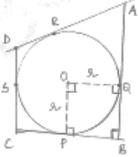∠OPB = ∠OQB = 90° = ∠B = ∠POQ
and PO = OQ
∴ POQB is a square PB = BQ = r
We know that
Tangents drawn from external point to circle are equal in length.
We know that
Tangents drawn from external point to circle are equal in length.
We know that
Tangents drawn from external point to circle are equal in length.
From A, AR = AQ ...(i)
From B, PB = QB ...(ii)
From C, PC = CS  ...(iii)
From D, DR = DS  ...(iv)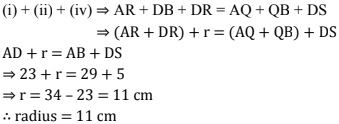20. In fig. there are two concentric circles with Centre O of radii 5 cm and 3 cm. From an external point P, tangents PA and PB are drawn to these circles if AP = 12 cm, find the tangent length of BP.
Solution
Given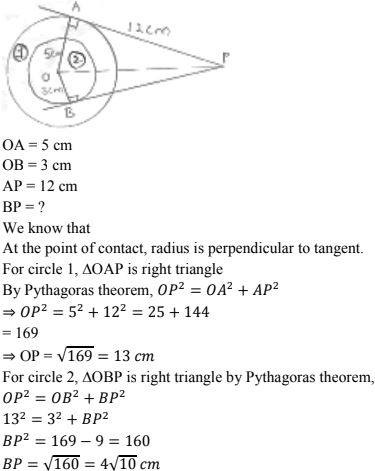21. In fig. AB is chord of length 16 cm of a circle of radius 10 cm. The tangents at A and B intersect at a point P. Find the length of PA.
Solution
Given length of chord AB = 16 cm.
Radius OB = OA = 10 cm.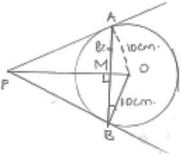Let line through Centre to point from where tangents are drawn be intersecting chord AB at M. we know that the line joining Centre to point from where tangents are drawn be intersecting chord AB at M. we know that the line joining Centre to point from where tangents are drawn bisects the chord joining the points on the circle where tangents intersects the circle.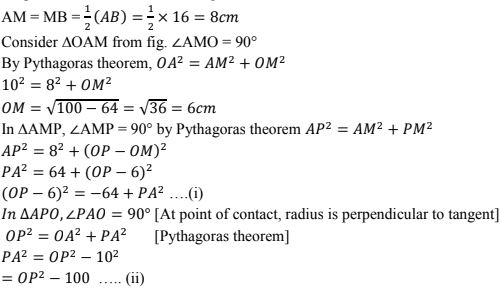22. In figure PA and PB are tangents from an external point p to the circle with centre O. LN touches the circle at M. Prove that PL + LM  = PN + MN
Solution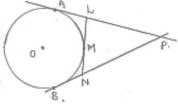Given
O is Centre of circle
PA and PB are tangents
We know that
The tangents drawn from external point to the circle are equal in length.
From point P, PA = PB
⇒ PL + AL = PN + NB ...(i)
From point L & N, AL = LM and MN = NB
Substitute in (i)
PL + LM = PN + MN
Hence proved.

23. In the fig. PO ⊥ QO. The tangents to the circle at P and Q intersect at a point T. Prove that PQ and OT are light bisectors of each other.
Solution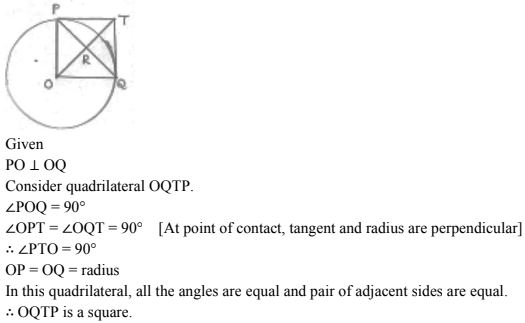24. In the fig two tangents AB and AC are drawn to a circle O such that ∠BAC = 120° . Prove that OA = 2AB.
Solution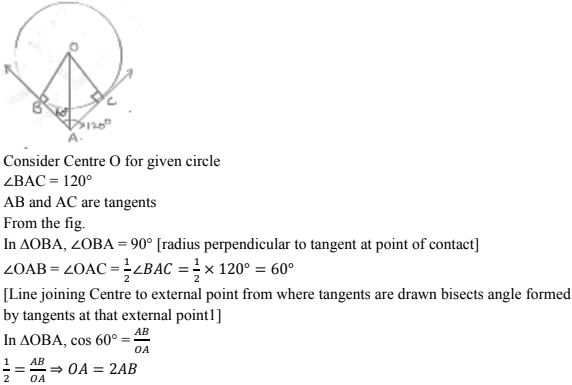25. In the fig. BC is a tangent to the circle with Centre O. OE bisects AP. Prove that ΔAEO ~ ΔABC.
Solution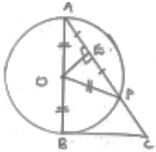Given
BC is tangent to circle
OE bisects AP, AE = EP
Consider ΔAOP

26. The lengths of three consecutive sides of a quadrilateral circumscribing a circle are 4 cm, 5 cm and 7 cm respectively. Determine the length of fourth side.
Solution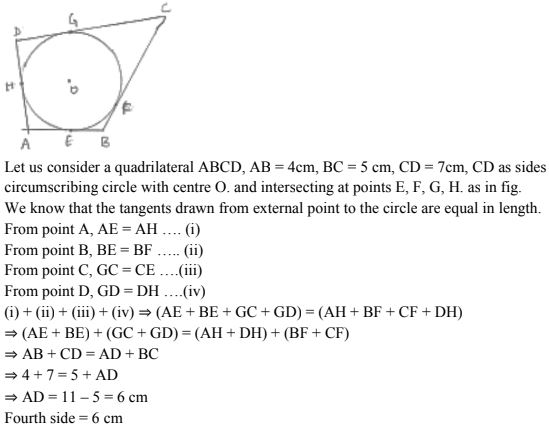27. In fig. common tangents PQ and RS to two circles intersect at A. Prove that PQ = RS.
Solution
Consider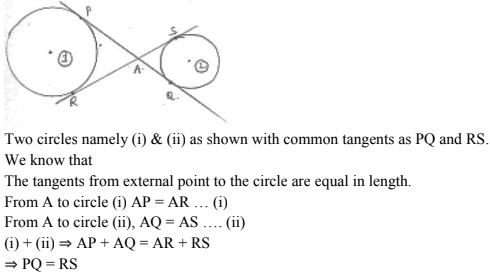28. Equal circles with centers O and O' touch each other at X. OO' produced to meet a circle with centre O' at A. AC is tangent to the circle whose centre is a O' D is perpendicular to AC. Find the value of DO'/CO
Solution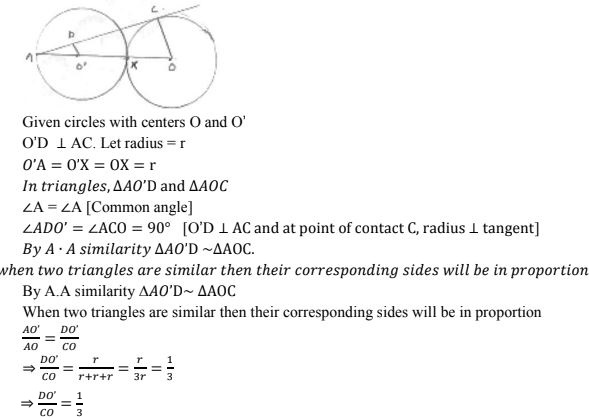29. In figure OQ : PQ = 3 : 4 and perimeter of ΔPDQ = 60 cm. determine PQ, QR and OP.
Solution